CBSE Sample Papers for Class 12 Computer Science Paper 2 are part of CBSE Sample Papers for Class 12 Computer Science. Here we have given CBSE Sample Papers for Class 12 Computer Science Paper 2.

## CBSE Sample Papers for Class 12 Computer Science Paper 2

 Board CBSE Class XII Subject Computer Science Sample Paper Set Paper 2 Category CBSE Sample Papers

Students who are going to appear for CBSE Class 12 Examinations are advised to practice the CBSE sample papers given here which is designed as per the latest Syllabus and marking scheme, as prescribed by the CBSE, is given here. Paper 2 of Solved CBSE Sample Paper for Class 12 Computer Science is given below with free PDF download Answers.

Time: 3 Hours
Maximum Marks: 70

General Instructions:

• All questions are compulsory within each Section.
• Programming Language in SECTION A : C++.

SECTION A

Question 1.
(a) Differentiate between the syntax error and runtime error Give one example of each.
(b) Which C++ header file(s) are essentially required to be included to run/execute the following C++ source code?

void main( )
{
int num;
cout<<"Enter the number:";
cin>>num;
if(abs(num)==num)
cout<<"Positive"<<endl;
}

(c) Observe the following C++ code carefully and rewrite the same after removing all the syntax error(s) present in the code. Ensure that you underline each correction in the code.
Note:
All the desired header files an. already included, which are required to run the code.
Correction should .not change the logic of the program.

Class Student
{
public:
int Student( ) { admno = 0; }
void input( )
{
}
void output( )
{
}
}
void Main( )
{
Student S;
input(S);
S.output( );
}

(d) Observe the following C++ code carefully and obtain the output, which will appear on the screen after the execution of it.
Note: All the desired header files are already included, which are required to run the code.

int main( )
{
char String[] = "Pointers and Strings";
cout<<*(&String )<<endl;
cout.write(String+5, 15)<<endl;
cout.write(String, 20)<<endl;
return( );
}

(e) Find the output of the following program:

class Weather
{
int Location, Temp, Humidity;
public:
Weatherdnt L=1)
{
Location = L;
Temp=15;
Humidity=65;
}
void Sun(int T)
{
Temp+=T;
}
void Rain(int H)
{
Humidity+=H;
}
void Check( )
{
cout<<Location<<":"<<Temp<<"&"<<Humidity<<"%"<<endl;
}
};
void main( )
{
Weather W, N(3);
W.Sun(5);
W.Check( );
N.Rain(10);
N.Sun(2);
N.Check( );
W.Rain(15);
W.Check( );
}

(f) In the following C++ program, what is the expected value of MyMarks from options (i) to (iv) given below? Justify your answer.

#include<stdio.h>
#include<iostream.h>
void main( )
{
randomize( );
int Marks[ ] = {99, 92, 94, 96, 93, 95}, MyMarks;
MyMarks = Marks[1+random(2)];
cout<<MyMarks<<endl;
}

(i) 99
(ii) 94
(iii) 96
(iv) 92

Question 2.
(a) What is a copy constructor? Illustrate with the help of C++ code.
(b) Answer the questions (i) and (ii) after going through the following class:

class Meeting
{
int Time;
public:
Meeting( ) //Function1
{
Time = 30;
cout<<"Meeting starts now"<<endl;
}
void Discussion(int) //Function2
{
cout<<”Discussion in the Meeting on"<<endl;
}
Meetingtint Duration) //Function3
{
Time = Duration;
cout<<"Meeting starts now"<<endl;
}
~Meeting( ) //Function4
{
cout<<"Vote for thanks"<>Percentage;
}
void DisplayData( )
{
cout<<setw(32)<<S_Name;
cout<<setw(3)<<Percentage<<endl;
}
int ReturnPercentage( )
{
return Percentage;
}
};

SECTION B

Question 5.
(a) Observe the following table and write the names of the most appropriate columns, which can be considered as (i) Degree and cardinality (ii) Primary key.(b) Write SQL queries for (i) to (iv) and find outputs for SQL queries (v) to (viii), which are based on the tables.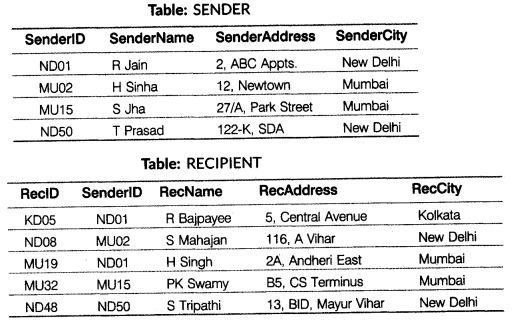(i) To display the name of all senders from Mumbai.
(ii) To display recipient details in ascending order of RecName.
(iii) To display Number of Recipients from each city.
(iv) To insert a new row in the table SENDER with the following data ‘KL05′, A.Tiwari’,’12-C Model Town’, ‘Kolkata’.

(v) SELECT DISTINCT SenderCity FROM SENDER;
(vi) SELECT A.SenderName, B.RecName FROM SENDER A, RECIPIENT B
WHERE A.SenderID = B.SenderlD AND B.RecCity = 'Mumbai';
(vii) SELECT RecName. RecAddress FROM RECIPIENT
WHERE RecCity NOT IN (’Mumbai’. ’Kolkata’);
(viii) SELECT RecID, RecName, SenderCity FROM RECIPIENT, SENDER
WHERE RECIPIENT.SenderID=SENDER.SenderID AND SenderCity='New Delhi';

Question 6.
(a) State and verify absorption law rising truth table.
(b) Draw the logic diagram of the expression $$\bar { X } \left( Y+Z \right) +\bar { X } \bar { Y }$$
(c) Write the Product of Sum (POS) form of the function H (U,V,W), truth table representation of H is as follows: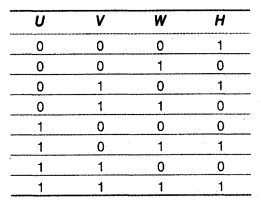(d) Obtain a simplified form for a Boolean expression using K-map.
F(w, x, y, z) = Σ (0, 1, 3, 5, 7, 9, 10, 11, 12, 13, 15)

Question 7.
(a) What is the use of PPP (Point to Point Protocol)?
(b) What are two types of modem?
(c) Differentiate between freeware and shareware.
(d) What is the importance of URL in networking?
(e) Akash Industry has set-up its new centre at Akansha Kunj and Web-based activities. The company compound has 4 buildings as shown in the diagram below:(i) Suggest a cable layout of connection between the buildings.
(ii) Suggest the most suitable place (i.e. Building) to house the server of this organisation with a suitable reason.
(iii) Suggest the placement of the following devices with justification
I. Internet Connecting Device/Modem
II. Switch
(iv) The organisation is planning to link its Buy Counter situated in various parts of the same city, which type of network out of LAN, MAN or WAN will be formed? Justify your answer.
(f) Categorises the following under GSM and CDMA:
(i) Switching System (SS)
(ii) Time Division Multiple Access (TDMA)
(iii) Frequency Division Multiple Access (FDMA)
(iv) Operation and Support System (OSS).
(g) What is the significance of term bandwidth? Give unit of bandwidth.

(a) Differences between syntax error and runtime error are as follows:(b) → cout( )
→ abs( )
(c) class Student
{
public:
Student() { admno = 0; }
void input( )
{
}
void output( )
{
}
};
void main( )
{
Student S;
S.input( );
S.output( );
}

(d) The output of the given program would be as given below:
i
ers and Strings
Pointers and Strings
(e) Output
1:20&65%
3:17&75%
1:20&80%
(f) The expected output will be:
(ii) 94
(iv) 92
Because random(2) will generate either 0 or 1. If it will generate 0 then the output will be (iv) 92. If it will generate 1 then the output will be (ii) 94.

(a) A copy constructor is a special constructor function that copies the contents of an object to another object, i.e. constructs an object using the data of another object of the same class.
e.g. To illustrate the copy constructor

#include<stdio.h>
#include<iostream.h>
class Counter
{
int num;
public:
Counter( ) { num=10; }
Counter(Counter &c) //Copy Constructor
{ num=c.num; }
void Print( )
{
cout<<num<<endl;
}
};
void main( )
{
Counter obj1;
cout<<"Object 1 variable value
obj1.Print( );
Counter obj2(obj1): //Calling copy constructor
cout<<"Object 2 variable value
obj2.Print( );
}

(b) (i) Concept illustrated by Function 1 and Function3 combinedly is called constructor overloading.
(ii) 1 time the message “Vote for thanks” will be displayed. Line4 is responsible to display the message “Vote for thanks”.

(c) class Dress
{
char CodeC20];
char Type;
int Size;
char Material;
float Price;
void Cal_Price( )
{
if(strcmp(Material,"Fabric")==0)
{
if (strcmpf Type, "Western" )==0)
Price=2500;
else if(strcmp(Type, "lndian")=0)
Price=2200;
}
else
{
if (strcmpdype, "Western" )==0)
Price=2500-0.20*2500;
else if (strcmp(type,"Indian")==0)
Price=2200-0.20*2200;
}
}
public:
Dress( )
{
strcpy(Code, "NOT ASSIGNED");
strcpy(type, "NOT ASSIGNED");
strcpy(Material,"NOT ASSIGNED");
Size=0;
Price=0;
}
void Input( )
{
cout<<"Enter dress code:"; cin>>Code;
cout<<"Enter type-(Western/Indian):"; cin>>Type;
cout<<"Enter size:"; cin>>Size;
cout<<"Enter material:"; cin>>Material;
Cal_Price( ):
}
void Dispiay( )
{
cout<<"Code:"<<Code<<endl;
cout<<"Type:"<<Type<<endl;
cout<<"Size:"<<Size<<endl;
cout<<"Material:"<<Material<<endl;
cout<<”Price:"<<Price<<endl;
}
};

(d) (i) The member functions inherited by class C are: getVal( ), dispval( ), getData( ), showData( ).
(ii) There is no base class of class B and subclass of class B is class C.
(iii) II. Multiple Inheritance
(iv) Data member, x, with private access scope is directly accessible only inside the class. Data member, z, with protected access scope is directly accessible within the class and by all the child classes of class B. Both the member function, with the public scope are accessible outside the class.

(a) void Reversearray(int A[ ], int n)
{
int tmp, i, j:
for(i=0, j=n-1; i<=j; i++, j--)
{
tmp = AC[i];
A[i] = A[j]:
A[j] = tmp:
}
for(i=0; i<n; i++)
cout<<A[i ]<<" ";
}

(b) Given,
Element size, W=4 bytes
Total rows, M=20
Total columns, N = 30
Ir = 0
Ic= 0
Column major
A[I][J] = B+W*[(I-Ir) + M*(J-Ic)]
A  = B + 4*[(2 – 0) + 20*(20 – 0)]
5000 = B + 4*[2 + 400]
5000 = B + 4*
5000 = 6+1608
B = 5000-1608 = 3392
A[I][J] = B+W*[(I-Ir) + M*(J-Ic)]
A =3392 + 4*[(5 – 0) + 20*(15 -0)]
= 3392 + 4*[5 + 300]
= 3392 + 4*
= 3392 + 1220
= 4612

(c) void Remove(MYNODE *Front, MYNODE *Rear)
{
MYNODE *Ptr = Front;
if(Ptr = NULL)
{
cout<<"Underflow!";
exit(1);
}
else if(Front == Rear)
{
delete Ptr;
Front = Rear = NULL;
else
{
delete Ptr;
}
}

(d) Given expression, (X-Y/(Z + U) *V)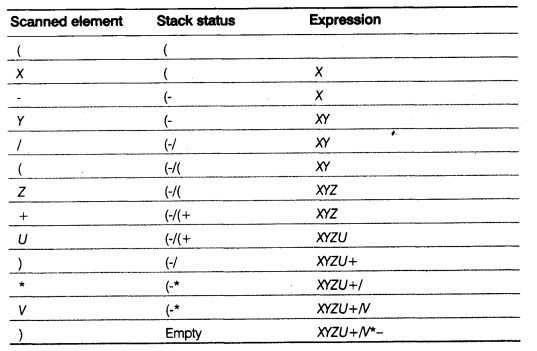Output XYZU + IV

(e) void SKIP(int A[ ], int M, int N)
{
int i, j;
for(i=0; i<=N-1; i++)
{
if(i%2==0)
{
j=0:
}
el se
{
j=1:
}
whi1e(j<=M-1)
{
cout<<A[i][j]<<"\t";
j=j+2;
}
}
}

(a) (i) Fi.seekp(-1*sizeof (f), ios::cur);
(ii) Fi .write((char*)&M, sizeof(M));

(b) #include<iostream.h>
#1nclude<fstream.h>
#include<ctype.h>
#include<string.h>
void main( )
{
int i = 0, Start = 0;
ifstream fin("Report.txt");
ofstream fout("Finerep.txt");
char word;
while(!fin.eof())
{
fin>>word;
if(Start==0)
{
word = toupper(word);
Start=1;
}
for(i=0; i<strlen(word); i++)
if (word[i ]= '.')
word[i+1] = toupper(word[i+1]);
fout<<word;
}
}
{
ifstream ifs;
ifs.open("STUDENT.DAT",ios::in | ios::binary);
if (!ifs)
{
cout<<"file does not exist";
exit(0);
}
Student St;
{
if(St.ReturnPercentage( )>75)
St.DispiayData( );
}
ifs.close( );
}

(a) (i) Degree=7
Cardinality = 6
(ii) Primary Key = CoachID

(b) (i) SELECT SenderName FROM SENDER
WHERE SenderCity = "Mumbai";
(ii) SELECT * FROM RECIPIENT ORDER BY RecName;
(iii) SELECT COUNT(*) AS "Number of Recipients", RecCity
FROM RECIPIENT GROUP BY RecCity;
(iv) INSERT INTO SENDER VALUES('KL05' , 'A.Tiwari', '12-C Model Town', 'Kolkata');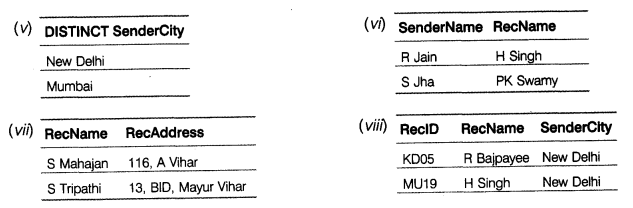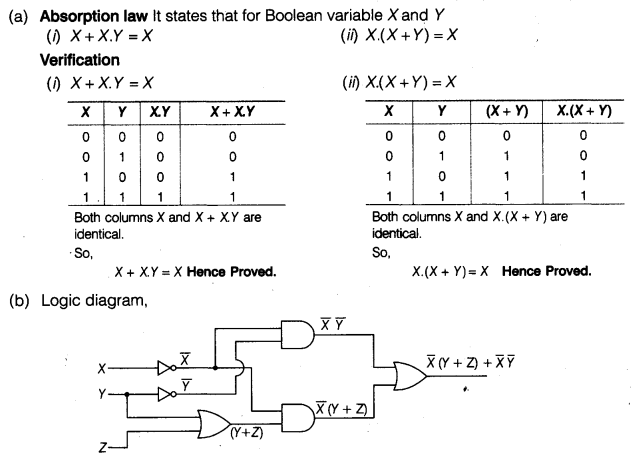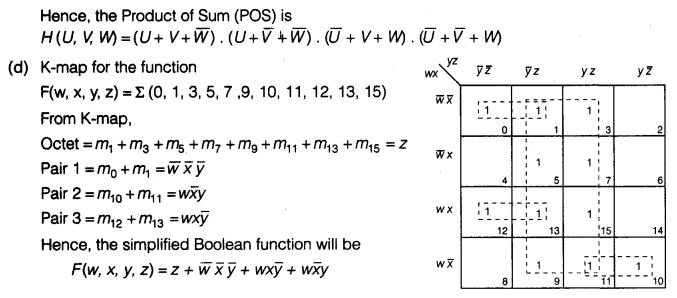(a) A point-to-point protocol is a communication protocol used to establish a direct connection between two nodes. It is the Internet standard for transmission of IP packets over serial lines.
(b) The modem comes in two varieties are as follows:
(i) Internal Modems These modems are fixed within a computer.
(ii) External Modems These modems are connected externally to a computer as other peripherals are connected.
(c) Freeware generally refers to the software, which is available for free of cost while shareware refers to the software, which is made available with a right to redistribute copies.
(d) URL stands for Uniform Resource Locator, each page that is created for Web browsing is assigned a URL that effectively serves as the page’s worldwide name or address. URL’s have three parts: the protocol, the DNS name of the machine on which the page is located and a local name uniquely indicating the specific page.
(e) (i) Cable Layout(ii) The most suitable place to house the server of this organization would be Sugar Mill Building, as this building contains the maximum number of computers thus, decreasing the cabling cost for most of the computers as well as will help to reduce the network traffic.
(iii) I. Sugar Mill Building because it has the maximum number of computers.
II. A switch will be needed in all the buildings to interconnect the group of cables from the different computers in each building.
(iv) MAN because MAN is the networks that link computer facilities within a city.
(f) GSM
(i) Switching System (SS)
(iv) Operation and Support System (OSS)
CDMA
(ii) Time Division Multiple Access (TDMA)
(iii) Frequency Division Multiple Access (FDMA)
(g) Bandwidth is the capability of a medium to transmit an amount of information over a unit of time. The bandwidth of a medium is generally measured in units of bits per second (bps) or more commonly in kilobits per second (Kbps).

We hope the CBSE Sample Papers for Class 12 Computer Science Paper 2 help you. If you have any query regarding CBSE Sample Papers for Class 12 Computer Science Paper 2, drop a comment below and we will get back to you at the earliest.